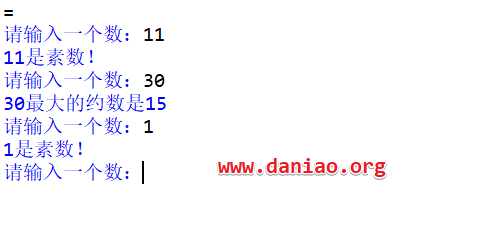# Python中丰富的else语句及简洁的with语句的学习笔记

2019年8月12日23:35:2823,575 3149字阅读10分29秒

Python中else语句的用法，不仅仅是和if来配对，也可以配合while和for循环来使用。

## else语句的用法：

#### 1）配合if语句

```if a>b:
print(a)
else:
```

#### 2）配合while和for循环

```def showMaxFactor(num):
count = num // 2
while count > 1:
if num % count == 0:
print('%d最大的约数是%d' % (num, count))
break
count -= 1
else:
print('%d是素数！' % num)

num = int(input('请输入一个数：'))
showMaxFactor(num)
```#### 3）try else语句

```try:
print('ABC')
except:
print('DEF')
else:
print('GHI')
finally:
print('JKL')
```

#try ....except...else 语句，当没有异常发生时，else中的语句将会被执行。所以结果为

ABC
GHI
JKL

#### 4）使用with语句固然方便，但如果出现异常的话，文件还会自动正常关闭吗？

`with语句会自动处理文件的打开和关闭，如果中途出现异常，会执行清理代码，然后确保文件自动关闭。`

5）你可以换一种形式写出下边的伪代码吗？

```with A() as a:
with B() as b:
suite
```

```with A() as a,with B() as b:
suite```

#### 5)使用 with 语句改写以下代码，让 Python 去关心文件的打开与关闭吧。0

```def file_compare(file1, file2):
f1 = open(file1)
f2 = open(file2)
count = 0 # 统计行数
differ = [] # 统计不一样的数量

for line1 in f1:
count += 1
if line1 != line2:
differ.append(count)

f1.close()
f2.close()
return differ

file1 = input('请输入需要比较的头一个文件名：')
file2 = input('请输入需要比较的另一个文件名：')

differ = file_compare(file1, file2)

if len(differ) == 0:
print('两个文件完全一样！')
else:
print('两个文件共有【%d】处不同：' % len(differ))
for each in differ:
print('第 %d 行不一样' % each)
```

with语句修改后代码如下：

#### 6）利用异常的原理，修改下面的代码使得更高效率的实现吗？t1sm

```print('|--- 欢迎进入通讯录程序 ---|')
print('|--- 1：查询联系人资料  ---|')
print('|--- 2：插入新的联系人  ---|')
print('|--- 3：删除已有联系人  ---|')
print('|--- 4：退出通讯录程序  ---|')

contacts = dict()

while 1:
instr = int(input('\n请输入相关的指令代码：'))

if instr == 1:
name = input('请输入联系人姓名：')
if name in contacts:
print(name + ' : ' + contacts[name])
else:
print('您输入的姓名不再通讯录中！')

if instr == 2:
name = input('请输入联系人姓名：')
if name in contacts:
print('您输入的姓名在通讯录中已存在 -->> ', end='')
print(name + ' : ' + contacts[name])
if input('是否修改用户资料（YES/NO）：') == 'YES':
contacts[name] = input('请输入用户联系电话：')
else:
contacts[name] = input('请输入用户联系电话：')

if instr == 3:
name = input('请输入联系人姓名：')
if name in contacts:
del(contacts[name])         # 也可以使用dict.pop()
else:
print('您输入的联系人不存在。')

if instr == 4:
break

print('|--- 感谢使用通讯录程序 ---|')
```•huahua 0

hhh好哦

•大鸟

额，私人的学习笔记，没想到还有人看